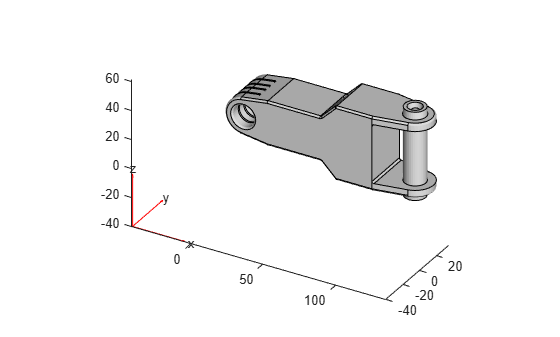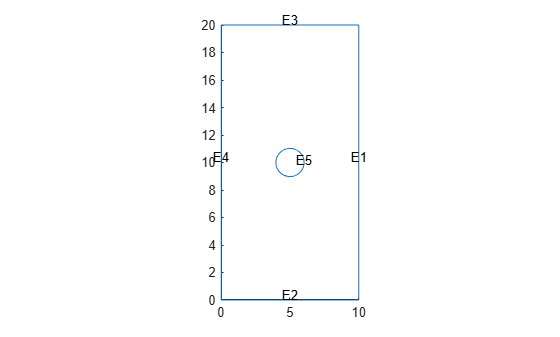Documentation

# importGeometry

Import geometry from STL data

## Syntax

``importGeometry(model,geometryfile)``
``gd = importGeometry(model,geometryfile)``

## Description

example

````importGeometry(model,geometryfile)` creates a geometry container from the specified STL geometry file, and includes the geometry in the `model` container.```
````gd = importGeometry(model,geometryfile)` also returns the geometry to the MATLAB® workspace.```

## Examples

collapse all

Import STL geometry into a PDE model.

Create a `PDEModel` container for a system of three equations.

`model = createpde(3);`

Import geometry into the container.

`importGeometry(model,'ForearmLink.stl');`

View the geometry with face labels.

`pdegplot(model,'FaceLabels','on')`Import a planar STL geometry into a PDE model. When importing a planar geometry, `importGeometry` converts it to a 2-D geometry by mapping it to the X-Y plane.

Create a `PDEModel` container.

`model = createpde;`

Import geometry into the container.

`importGeometry(model,'PlateHolePlanar.stl')`
```ans = DiscreteGeometry with properties: NumCells: 0 NumFaces: 1 NumEdges: 5 NumVertices: 5 ```

View the geometry with edge labels.

`pdegplot(model,'EdgeLabels','on')`## Input Arguments

collapse all

Model object, specified as a `PDEModel` object, `ThermalModel` object, or `StructuralModel` object.

Example: `model = createpde(1)`

Example: `thermalmodel = createpde('thermal','steadystate')`

Example: `structuralmodel = createpde('structural','static-solid')`

Path to STL file, specified as a character vector or a string scalar ending with the file extension `.stl` or `.STL`.

Example: `'../geometries/Carburetor.stl'`

Data Types: `char` | `string`

## Output Arguments

collapse all

Geometry description, returned as a `DiscreteGeometry` object. See DiscreteGeometry Properties for details.

## Limitations

• `importGeometry` does not allow you to import a multidomain 3-D geometry unless all of its subdomains are separate. In other words, the subdomains of the geometry cannot have any common points. Because of this limitation, you cannot import nested 3-D geometries directly. As a workaround, you can import a mesh and then create a multidomain geometry from the mesh by using the `geometryFromMesh` function.

## Tips

• STL format approximates the boundary of a CAD geometry by a collection of triangles, and `importGeometry` reconstructs the faces and edges from this data. Reconstruction from STL data is not precise and can result in a loss of edges and, therefore, the merging of adjacent faces. Typically, lost edges are the edges between two adjacent faces meeting at a small angle, or smooth edges bounding blend surfaces. Usually, the loss of such edges does not affect the analysis workflow.## See Also

### Topics

#### Introduced in R2015a

##### SupportGet trial now